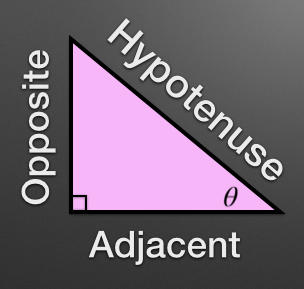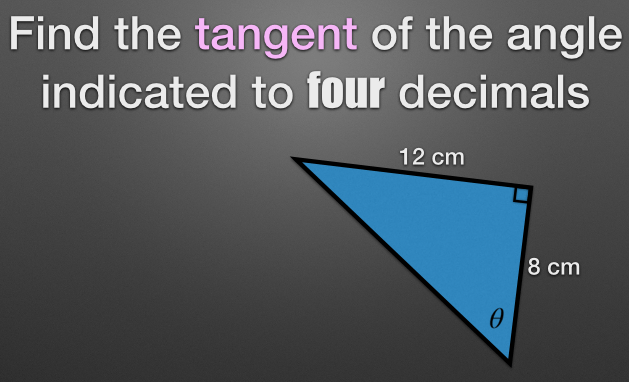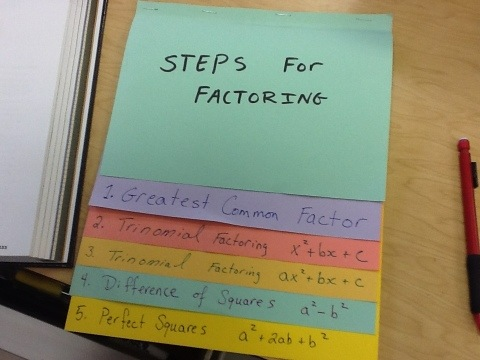# Introducing Trig through Slope

Here is our lesson today to introduce trigonometry for the first time. We had spent a few days with solving problems with similar triangles. We are spiralling and have done  lots of work recently using slope and the distance formula to classify triangles. I wanted to capitalize on that familiarity with slope to introduce the tangent ratio for the first time.

We started with this….againMost students like last time chose A and their reason was it was less steep. So I asked “How much less?” “How do we measure that?”……SLOPE was the response and they calculated the slopes to verify.

I stressed supreme accuracy and added “Try to create a size of triangle you think no one else will make”……I had them measure their rise and run and enter them in this table on the board.I also kept a running table in Desmos…As more students added their triangles I could hear them say, “I bet all the slopes should be the same” , “They’re all similar triangles” We took a moment to discuss similarities and make it clear we all have similar triangles and that the ratio between the rise and the run should all be the same. We also discussed why some of our triangles did not have a slope of 1.7. I had them repeat the process with an angle of 45 degrees.I said out loud that MY slope ratio was 1….and I could see all their heads bobbing up and down….”Yep, we got 1 too”.

Next….I asked them again to create an angle/triangle (Had them keep the same orientation of the triangle as I did in my diagram) that no one else would.

Measure the rise and the run, then calculate your slope. Keep your triangle and slope hidden, especially from ME.Keeping their angles and ratios hidden from me I said…”When I point to you tell me your angle….and I’ll magically tell you your slope” Cue the Oooohs and aaaahs.

I played up the magic bit. I held my calculator up to shield the screen from them.

I pointed at one student they told me “34 degrees”. I punched on my calculator mysteriously and said…”0.67.” The student yelled out….”Hey that’s right”. I went around the room pointing at students and telling them their slopes (ratios). I could see it on their faces, they wanted to know how I was doing this……Boom Let’s talk about Trigonometry.

So I said:

“In math we have these things called functions….they’re like black boxes that take an input and do some number crunching and spit out an output. One function you have used already is the square root function. You give the function 9 and it spits out 3. We math people use a symbol for this function so we all know what is going on. There is another function that will calculate the slope of a right triangle if you give it the angle. So we could write something like this “(I used one of the students angles).“This is what I was doing when you gave me your angles….I was using the function to calculate your ratio between rise and run. But we don’t usually use the term slope when we talk about right triangles. We use fancy words.” I had them draw a right triangle in their notes and we labeled it with Hypotenuse, opposite and adjacent.“Instead of using a slope function…..we use the word TANGENT. And instead of using the word rise we use the word OPPOSITE and instead of run we use ADJACENT. So we can write this tangent function equal to the rise/run = opp/adj.”“And we math people don’t like to write too much so we really use this version.”Then we practiced using the tangent button on our calculators. They pretended to be the magicians and checked each others ratios. We practiced using the inverse tangent button to find angles.

Once we were comfortable we moved into writing the ratio and finding the angle out. We also used this example to write the tangent ratio of the other angle.and then one more for lengths:Since we are spiralling I gave them the homework set (Mary Bourassa Style) to work on….here.

Tomorrow I’ll introduce the Sine and Cosine function.

Using slope here to introduce trig allows us to take something familiar and make something new. Students could see the progression happen and not have trig just thrown at them.

Would to love to hear your thoughts on this. How do you introduce trig?# Grade 10 Expanding Factoring Assignment!

Grade 10’s … get your Expanding Factoring Assignment here if you didn’t get one in class.
Factoring Assignment

Don’t forget about part 3 of the CSI Assignment too!

# Factoring Foldable!

While we learn factoring techniques we will keep examples and notes in our Factoring Foldable.

As we learn each new technique students will add those examples to the foldable.

Here are some samples.Update [Oct 28 2014] – Students are loving this foldable……some even enough to keep it year after year. Here is one of my NOW returning grade 12 students who has kept her foldable for the last three years.Other foldables:

Solving Linear Systems

Slope Foldable